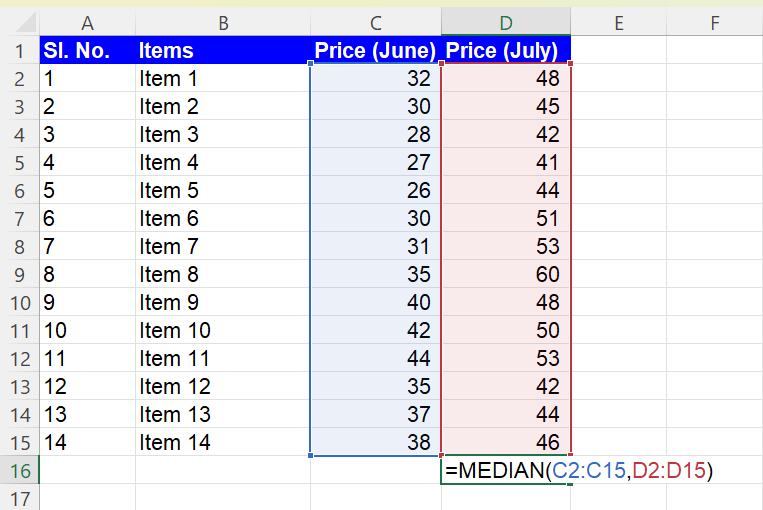Excel Formulas

# How to Use MEDIAN Function in Excel

## The MEDIAN formula in Excel

The MEDIAN function in Excel is used to arrive at the median value among a set of selected values. To explain further, the MEDIAN formula gives the middlemost value when the selected values are arranged in sequence (ascending or descending order). It is useful when you want to find the central tendency of a group of numbers, especially when the data contains outliers that can skew the mean (average) of the dataset.

## Understanding the MEDIAN function syntax in Excel

``=MEDIAN(number1, [number2], ...)``

In the above syntax, the median of the numbers selected will be displayed.

For example: if 4 is number 1, 6 is number 2 and 2 is number 3, the formula =MEDIAN(4,6,2) will deliver the median value to be 4.

## Using the MEDIAN formula in Excel

Here are some situations where you might use the MEDIAN formula in Excel:

1. Inspecting salary data to determine the middle salary in a group of employees
2. Examining raw material costs to determine the median raw material cost among months in a year
3. Analyzing sales data to determine the median sales amount for a group of products
4. Investigating test scores to determine the median score for a group of students

In general, the MEDIAN formula is useful anytime you want to find the value that is in the middle of a range of numbers. It can be a more accurate measure of central tendency in datasets that contain extreme values, and it is often used in conjunction with other statistical measures like mean, mode, and standard deviation to provide a more complete picture of the data.

## Inserting the MEDIAN function in Excel

Step 1: Type “=MEDIAN(“ in the cell where you wish to calculate the median value

Step 2: Select the values one after the other by separating them using “,” (commas). You can also select arrays of data as shown in the image below, where you are calculating the median of the prices charged in the months of June and July.Picture showing the MEDIAN function used to select two arrays of data in Excel

Step 3: Press the “ENTER” key

NOTE: The MEDIAN formula takes into account zeros in the selected data set. Hence, in case your data contains missing data points, make sure that it is not represented using a zero. You can instead use any form of free text such as “missing” or “ NA” or any other text comment to represent the missing data points.

## Analyze your live financial data in a snap in Google Sheets

Are you learning this formula to visualize financial data, build a financial model, or conduct financial analysis? In that case, LiveFlow may help you automate manual workflows, update numbers in real-time, and save time. You can access various financial templates on our website, from the simple Income Statement to Multi-Currency Consolidated Financial Statement. Are you interested in this product but are an Excel user? That’s not a problem at all. You can connect Google Sheets to Excel quickly.

You can learn about other Excel and Google Sheets formulas and tips that are not mentioned here on this page: LiveFlow‘s How to Guides

Learn how to do this step-by-step in the video below 👇

## Do you need personal help?

Our team of real people are here to help you any time between 9am and 10pm EST.Email us at: help@liveflow.ioCall us at +1 (415) 650-1711